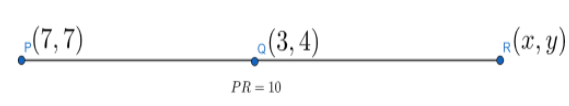QuestionAnswers

# If P,Q,R are collinear points such that, $P(7,7)$, $Q(3,4)$ and $PR=10$, find $R$.

Hint: Assume the co-ordinates of $R$ as $(x,y)$ and as it is given that $P,Q,R$ are collinear points. Apply distance condition of collinear points which is if $P,Q,R$ are three collinear points then they must satisfy $PQ+QR=PR$ and get one equation. Apply distance formula which states that if $A({{x}_{1}},{{y}_{1}})$ and $B({{x}_{2}},{{y}_{2}})$ are two points then the distance $AB=\sqrt{{{({{x}_{2}}-{{x}_{1}})}^{2}}+{{({{y}_{2}}-{{y}_{1}})}^{2}}}$ and get the second equation by using $PR=10$ , solve these two equations and get the co-ordinates of $R$.

Complete step-by-step solution:
It is given in the question that $P, Q, R$ are collinear points.
Collinear means lying on the same line which means that $P, Q, R$ lies on the same straight line.
Let us suppose that the co-ordinates of $R$ is $(x,y)$. Now, we have $P(7,7)$, $Q(3,4)$, $R(x,y)$ and also $PR=10$.We have to use distance formula in order to find $PQ,QR,PR$. So, according to distance formula if $A({{x}_{1}},{{y}_{1}})$ and $B({{x}_{2}},{{y}_{2}})$ then $AB=\sqrt{{{({{x}_{2}}-{{x}_{1}})}^{2}}+{{({{y}_{2}}-{{y}_{1}})}^{2}}}$.
Now, $P,Q,R$ are three collinear points then they must satisfy,
$\therefore PQ+QR=PR$
Using distance formula, we get
$\Rightarrow \sqrt{{{(7-3)}^{2}}+{{(7-4)}^{2}}}+\sqrt{{{(3-x)}^{2}}+{{(4-y)}^{2}}}=10$
$\Rightarrow \sqrt{16+9}+\sqrt{{{(3-x)}^{2}}+{{(4-y)}^{2}}}=10$
$\Rightarrow 5+\sqrt{{{(3-x)}^{2}}+{{(4-y)}^{2}}}=10$
$\Rightarrow \sqrt{{{(3-x)}^{2}}+{{(4-y)}^{2}}}=5$
Squaring both sides, we get
$\Rightarrow {{(3-x)}^{2}}+{{(4-y)}^{2}}=25$
$\Rightarrow {{x}^{2}}+{{y}^{2}}-6x-8y+25=25$
Cancelling 25 from both sides, we get
$\Rightarrow {{x}^{2}}+{{y}^{2}}=6x+8y...................(1)$
Also, it is given that $PR=10$, using this we get
$\therefore PR=10$
$\Rightarrow \sqrt{{{(7-x)}^{2}}+{{(7-y)}^{2}}}=10$
Squaring both sides, we get
$\Rightarrow {{(7-x)}^{2}}+{{(7-y)}^{2}}=100$
$\Rightarrow {{x}^{2}}+{{y}^{2}}-14x-14y+98=100$
Putting the value of ${{x}^{2}}+{{y}^{2}}$ from equation (1), we get
$\Rightarrow 6x+8y-14x-14y=100-98$
$\Rightarrow -8x-6y=2$
Now, expressing $y$ in terms of $x$, we get
$\Rightarrow y=\dfrac{-(2+8x)}{6}=\dfrac{-(1+4x)}{3}..................(2)$
Putting the value of $y$ from equation (2) in equation (1), we get
$\Rightarrow {{x}^{2}}+{{\left( \dfrac{-(1+4x)}{3} \right)}^{2}}=6x+\dfrac{-(1+4x)}{3}\times 8$
$\Rightarrow \dfrac{9{{x}^{2}}+1+8x+16{{x}^{2}}}{9}=\dfrac{18x-8-32x}{3}$
$\Rightarrow 25{{x}^{2}}+8x+1=3(-14x-8)$
$\Rightarrow 25{{x}^{2}}+8x+1+42x+24=0$
$\Rightarrow 25{{x}^{2}}+50x+25=0$
$\Rightarrow 25({{x}^{2}}+2x+1)=0$
$\Rightarrow {{(x+1)}^{2}}=0$
$\Rightarrow x+1=0\Rightarrow x=-1$
Now, putting the value of $x$ in equation (2), we get
$\Rightarrow y=\dfrac{-(1+4\times -1)}{3}$
$\Rightarrow y=\dfrac{3}{3}=1$
So, $x=-1$ and $y=1$.
Hence the co-ordinates of $R$ is $(-1,1)$.

Note: This question is simple and there are many other alternative ways to solve it but the tricky part is to choose which method to solve the question. There are three different conditions of collinearity as there is slope condition, distance condition, and area condition. As in the other two conditions distances are not involved, only the distance condition involves the use of distances. In the question, distance is mentioned so we choose that method to solve it. Hence, students should carefully read the question and choose which method is suitable to solve the question according to the values given.